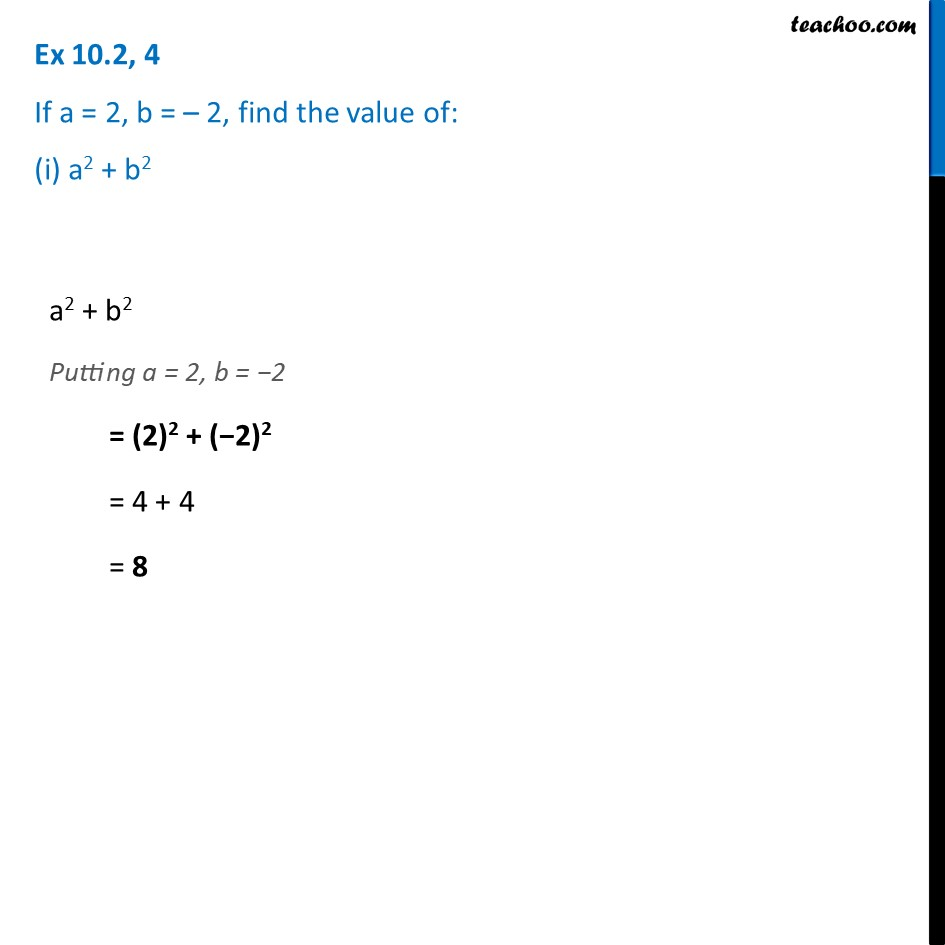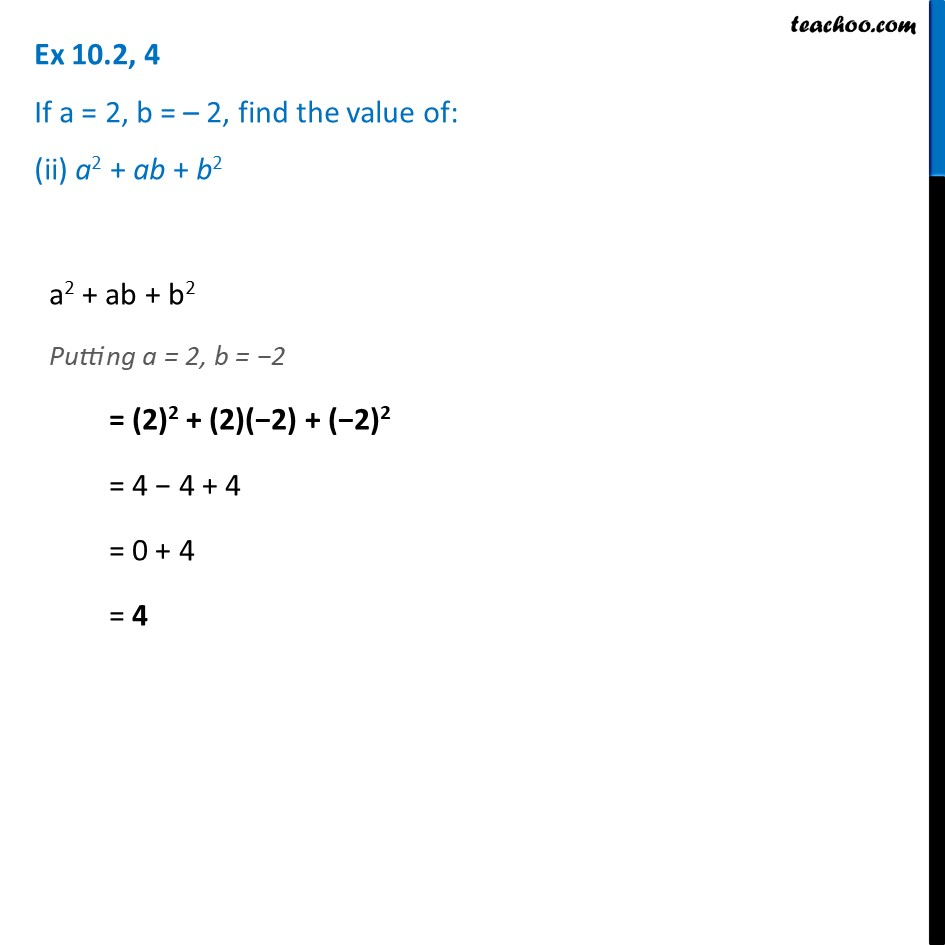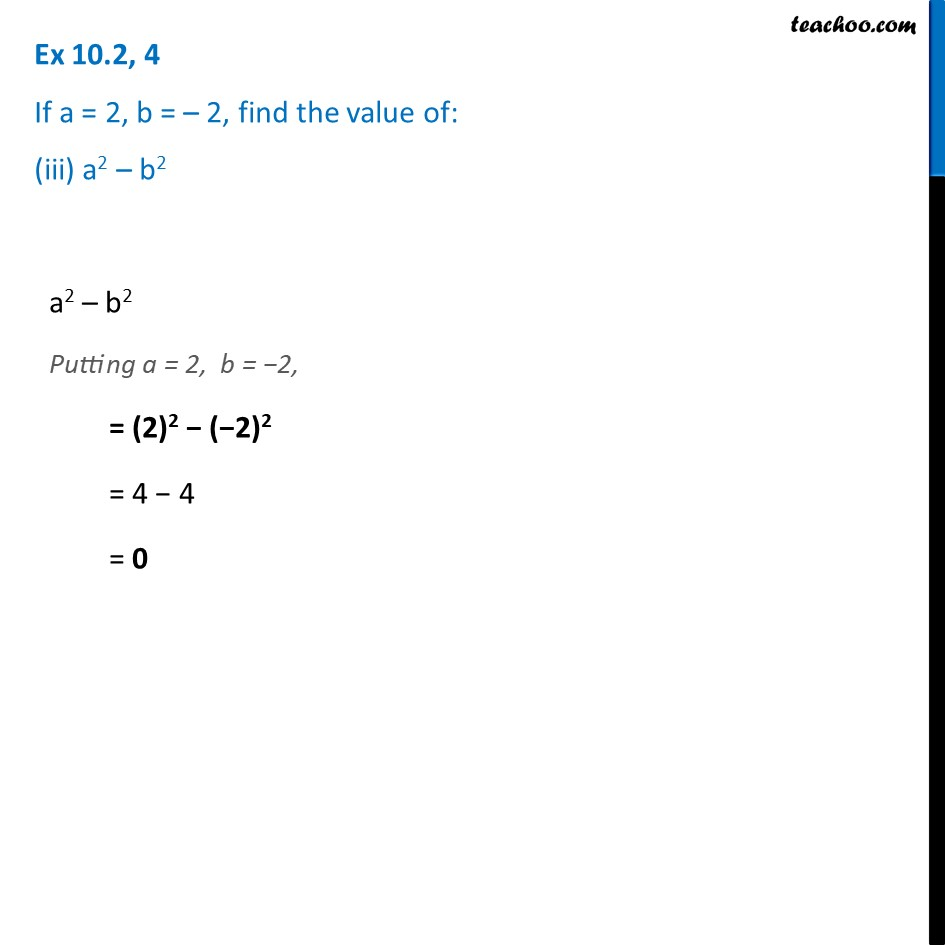Ex 10.2

Chapter 10 Class 7 Algebraic Expressions
Serial order wiseLearn in your speed, with individual attention - Teachoo Maths 1-on-1 Class

### Transcript

Ex 10.2, 4 If a = 2, b = – 2, find the value of: (i) a2 + b2a2 + b2 Putting a = 2, b = −2 = (2)2 + (−2)2 = 4 + 4 = 8 Ex 10.2, 4 If a = 2, b = – 2, find the value of: (ii) a2 + ab + b2a2 + ab + b2 Putting a = 2, b = −2 = (2)2 + (2)(−2) + (−2)2 = 4 − 4 + 4 = 0 + 4 = 4 Ex 10.2, 4 If a = 2, b = – 2, find the value of: (iii) a2 – b2a2 – b2 Putting a = 2, b = −2, = (2)2 − (−2)2 = 4 − 4 = 0